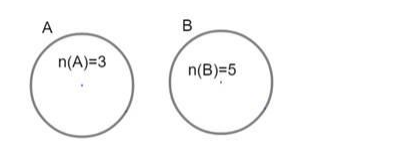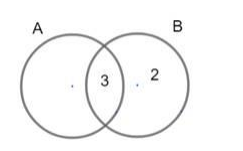# If n(A) = 3 and n(B) = 5, find:

Question:

If $n(A)=3$ and $n(B)=5$, find:

(i) The maximum number of elements in $A \cup B$,

(ii) The minimum number of elements in $A \cup B$.

Solution:

Number of elements in set A n(A) = 3 and number of elements in set B n(B) =

5

The number of elements in $A \cup B$ is $n(A \cup B)$.

i) Now for elements in $\mathrm{A} \cup \mathrm{B}$ to be maximum, there should not be any intersection between both sets that is $A$ and $B$ both sets must be disjoint sets as shown.Hence the number of elements in $A \cup B$ is $n(A \cup B)=n(A)+n(B)$

$\Rightarrow \mathrm{n}(\mathrm{A} \cup \mathrm{B})=3+5$

$\Rightarrow \mathrm{n}(\mathrm{A} \cup \mathrm{B})=8$

Hence maximum number of elements in $A \cup B$ is 8

ii) Now for a number of elements in $\mathrm{A} \cup \mathrm{B}$ to be minimum, there should be an ntersection between sets $A$ and $B$ so that some elements are common

The count will be minimum when all the elements from set $A$ are also in set $B$ the reverse are not possible because $n(A) Hence if the 3 elements of$A$are in the intersection of$A$and$B$, then the number of elements only in$B$will be 2 because$n(B)=5$Visually it is represented asAs seen from the figure the number of elements in$A \cup B$is 5 hence minimum number of elements in$A \cup B=5\$